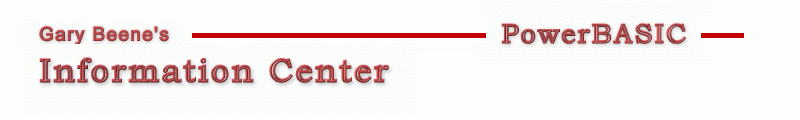## Custom UDT Sort

Category: Arrays

Date: 03-28-2012

'This code was written to compile in PBWin10. To compile in PBWin9,
'replace CALL with USING in the Array Sort statements (2 places).

'Compilable Example:
#Compiler PBWin 10
#Compile EXE
#Dim All
#Include "Win32API.inc"

Type myData
s As String * 10
l As Long
End Type

Global hDlg As Dword
Global D() As myData

Function PBMain() As Long
Dialog New Pixels, 0, "UDT Sort Test",300,300,200,100, %WS_OverlappedWindow To hDlg
FakeData
Control Add Button, hDlg, 100,"Push", 50,10,100,20
Dialog Show Modal hDlg Call DlgProc
End Function

CallBack Function DlgProc() As Long
Local i As Long, temp\$
If Cb.Msg = %WM_Command And Cb.Ctl = 100 And Cb.CtlMsg = %BN_Clicked Then
Dim X(UBound(D)) As myData At VarPtr(D(0))

temp\$ = "Descending Numeric:" + \$CrLf
Array Sort X(), Call SortNumeric()   'by value of long
For i = 0 To UBound(D) : temp\$ = temp\$ + \$CrLf + D(i).s : Next i : ? temp\$

temp\$ = "Descending Length:" + \$CrLf
Array Sort X(), Call SortLength()   'by length of .S
For i = 0 To UBound(D) : temp\$ = temp\$ + \$CrLf + D(i).s : Next i : ? temp\$
End If
End Function

Sub FakeData
ReDim D(3)
D(0).s = "ax-0"
D(1).s = "bxxxxx-1"
D(2).s = "cxxxx-2"
D(3).s = "dxx-3"
D(0).L = 0
D(1).L = 1
D(2).L = 2
D(3).L = 3
End Sub

Function SortNumeric(A As myData, B As myData) As Long
' -1 if 1st should precede 2nd : +1 if 2nd should precede 2nd
' this routine sorts on the .L (Long) element of the UDT
Function = IIf(A.L > B.L,-1,+1)        'descending numeric
End Function

Function SortLength(A As myData, B As myData) As Long
' -1 if 1st should precede 2nd : +1 if 2nd should precede 2nd
' this routine sorts on the .S (String*10) element of the UDT
Function = IIf(Len(Trim\$(A.s)) > Len(Trim\$(B.s)),-1,+1)   'descending alpha
End Function

'gbs_00894
'Date: 03-10-2012

created by gbSnippets
http://www.garybeene.com/sw/gbsnippets.htm